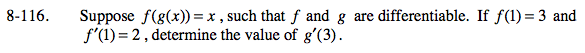### Home > CALC > Chapter 8 > Lesson 8.3.2 > Problem8-116

8-116.Recall that f(g(x)) = x is the definition of inverse functions. In other words, you are being told that f(x) and g(x) are inverses.

Inverse functions have reciprocal slopes at corresponding (x, y)→(y, x) values.

$\text{For example, if }f(x)\text { has coordinate point (2, 3) and }f'(2)=\frac{4}{5},\text{ then }g'(3)=\frac{5}{4}.$